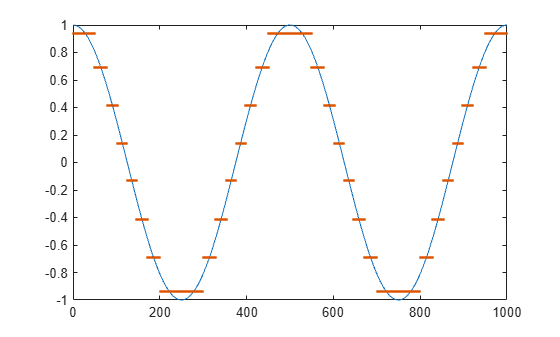# lloyds

Optimize quantization parameters using Lloyd algorithm

## Syntax

``[partition,codebook] = lloyds(training_set,initcodebook) ``
``[partition,codebook] = lloyds(training_set,len)``
``[partition,codebook] = lloyds(___,tol)``
``[partition,codebook,distor] = lloyds(___)``
``````[partition,codebook,distor,reldistor] = lloyds(___)``````

## Description

````[partition,codebook] = lloyds(training_set,initcodebook) ` optimizes the scalar quantization parameters `partition` and `codebook` for the training data in the vector `training_set`. `initcodebook` is the initial guess of the codebook values.```

example

````[partition,codebook] = lloyds(training_set,len)` is the same as the first syntax, except that the scalar argument `len` indicates the size of the vector `codebook`. This syntax does not include an initial codebook guess.```
````[partition,codebook] = lloyds(___,tol)` specifies the relative distortion tolerance,`tol`, in addition to any input argument combination in the previous syntaxes.```
````[partition,codebook,distor] = lloyds(___)` additionally returns the final mean square distortion, `distor`.```
``````[partition,codebook,distor,reldistor] = lloyds(___)``` additionally returns a value that depends on how the algorithm terminates, `reldistor`. ```

## Examples

collapse all

Create a sinusoidal signal to use as training data.

```t = 0:1000; training_set = sin(t*pi/500);```

Set a codeword length of eight. This length corresponds to transmission via a 3-bit channel.

`len = 8;`

Optimize the quantization parameters. Use the optimized parameters to quantize a different sinusoidal signal. Plot the signal and the quantized signal.

```[partition,codebook] = lloyds(training_set,len); signal = cos(t*pi/250); [index,quants] = quantiz(signal,partition,codebook); plot(t,signal,t,quants,'.')```## Input Arguments

collapse all

Training data, specified as a vector.

Note

The function optimizes for the data in `training_set`. For best results, `training_set` should be similar to the data that you plan to quantize.

Data Types: `double` | `single`

Initial codebook guess, specified as vector. The length of the vector must be at least 2. Specify this input to provide an initial value for the iterative process described in Algorithms.

Data Types: `double` | `single`

Initial codebook length, specified as a positive integer. Specify this input when you want the function to generate an initial value for the iterative process described in Algorithms.

Data Types: `double` | `single`

Relative distortion tolerance, specified as a scalar. The Lloyd algorithm terminates once the relative change in distortion between iterations is less than this value.

Data Types: `double` | `single`

## Output Arguments

collapse all

Distinct endpoints of different ranges, returned as a row vector. This output defines several contiguous, nonoverlapping ranges of values within the set of real numbers. The length of this vector is one less than the length of `codebook`. The data type is the same as that of `training_set`.

Quantization value for each partition, returned as a row vector. This output prescribes a common value for each partition in a scalar quantization. The length of this vector is equal to the length of `initcodebook` or the value of `len`, depending on which input you specify. The data type is the same as that of `training_set`.

Final mean square distortion, returned as a positive scalar. The function calculates the mean square distortion at each step of the Lloyd algorithm.

Final relative change in mean square distortion, returned as a positive scalar. If the algorithm terminates because of the first condition in Algorithms, `reldistor` is the relative change in distortion between the last two iterations. For the second condition, `reldistor` is equal to `distor`.

## Algorithms

The `lloyds` function uses an iterative process to minimize the mean square distortion. Optimization processing ends when either:

• The relative change in distortion between iterations is less than the value of `tol`.

• The distortion is less than `eps*max(training_set)`, where `eps` is the MATLAB® floating-point relative accuracy.

 Lloyd, S.P., “Least Squares Quantization in PCM,” IEEE Transactions on Information Theory, Vol. IT-28, March, 1982, pp. 129–137.

 Max, J., “Quantizing for Minimum Distortion,” IRE Transactions on Information Theory, Vol. IT-6, March, 1960, pp. 7–12.